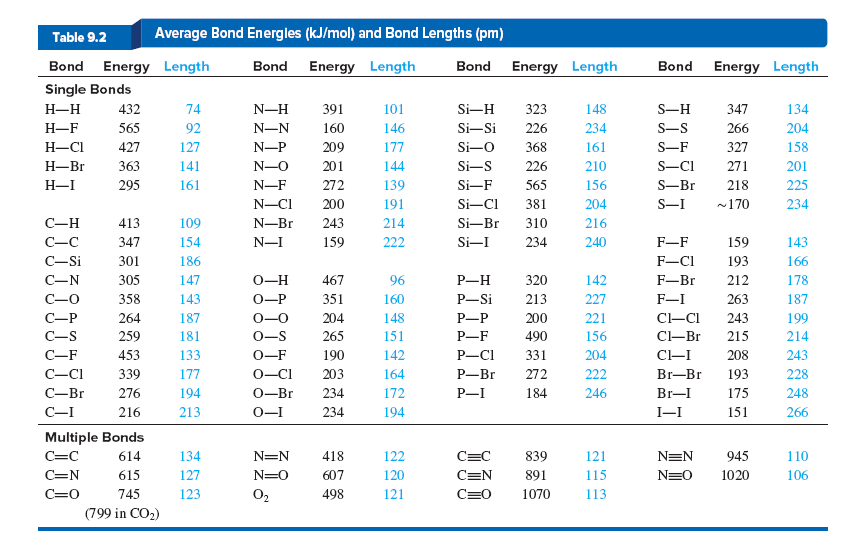# Problem: Dimethyl ether (CH3OCH3) and ethanol (CH3CH2OH) are constitutional isomers (see Table 3.3).(a) Use following table to calculate ΔH°rxn for the formation of each compound as a gas from methane and oxygen; water vapor also forms.

###### FREE Expert Solution

(A) For dimethyl ether (CH3OCH3) the reaction would be:

2 CH4 + O2 → CH3OCH3 + H2O

Drawing the Lewis structure of each, the reaction would be:

91% (163 ratings)###### Problem Details

Dimethyl ether (CH3OCH3) and ethanol (CH3CH2OH) are constitutional isomers (see Table 3.3).

(a) Use following table to calculate ΔH°rxn for the formation of each compound as a gas from methane and oxygen; water vapor also forms.